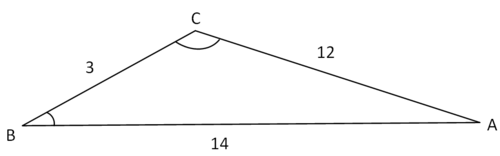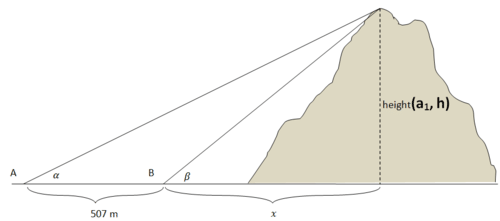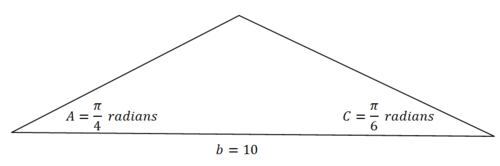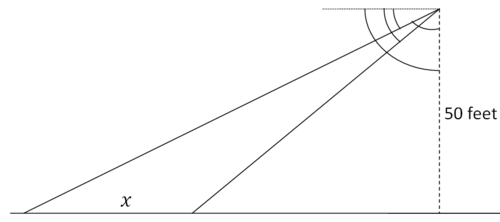# 4.8: Applications of Basic Triangle Trigonometry

$$\newcommand{\vecs}{\overset { \rightharpoonup} {\mathbf{#1}} }$$ $$\newcommand{\vecd}{\overset{-\!-\!\rightharpoonup}{\vphantom{a}\smash {#1}}}$$$$\newcommand{\id}{\mathrm{id}}$$ $$\newcommand{\Span}{\mathrm{span}}$$ $$\newcommand{\kernel}{\mathrm{null}\,}$$ $$\newcommand{\range}{\mathrm{range}\,}$$ $$\newcommand{\RealPart}{\mathrm{Re}}$$ $$\newcommand{\ImaginaryPart}{\mathrm{Im}}$$ $$\newcommand{\Argument}{\mathrm{Arg}}$$ $$\newcommand{\norm}{\| #1 \|}$$ $$\newcommand{\inner}{\langle #1, #2 \rangle}$$ $$\newcommand{\Span}{\mathrm{span}}$$ $$\newcommand{\id}{\mathrm{id}}$$ $$\newcommand{\Span}{\mathrm{span}}$$ $$\newcommand{\kernel}{\mathrm{null}\,}$$ $$\newcommand{\range}{\mathrm{range}\,}$$ $$\newcommand{\RealPart}{\mathrm{Re}}$$ $$\newcommand{\ImaginaryPart}{\mathrm{Im}}$$ $$\newcommand{\Argument}{\mathrm{Arg}}$$ $$\newcommand{\norm}{\| #1 \|}$$ $$\newcommand{\inner}{\langle #1, #2 \rangle}$$ $$\newcommand{\Span}{\mathrm{span}}$$$$\newcommand{\AA}{\unicode[.8,0]{x212B}}$$

Deciding when to use SOH, CAH, TOA, Law of Cosines or the Law of Sines is not always obvious. Sometimes more than one approach will work and sometimes correct computations can still lead to incorrect results. This is because correct interpretation is still essential.

If you use both the Law of Cosines and the Law of Sines on a triangle with sides 4, 7, 10 you end up with conflicting answers. Why?

## Trigonometry Applications

When applying trigonometry, it is important to have a clear toolbox of mathematical techniques to use. Some of the techniques may be review like the fact that all three angles in a triangle sum to be $$180^{\circ}$$, other techniques may be newer like the Law of cosines. Take a look at all the tools you have in your toolbox to solve applications with trigonometry.

### Toolbox

• The three angles in a triangle sum to be $$180^{\circ}$$.
• There are $$360^{\circ}$$ in a circle and this can help us interpret negative angles as positive angles.
• The Pythagorean Theorem states that for legs $$a, b$$ and hypotenuse $$c$$ in a right triangle, $$a^{2}+b^{2}=c^{2}$$
• The Triangle Inequality Theorem states that for any triangle, the sum of any two of the sides must be greater than the third side.
• The Law of cosines: $$c^{2}=a^{2}+b^{2}-2 a b \cos C$$
• The Law of sines: $$\frac{a}{\sin A}=\frac{b}{\sin B}$$ or $$\frac{\sin A}{a}=\frac{\sin B}{b}$$ (Be careful for the ambiguous case)
• SOH CAH TOA is a mnemonic device to help you remember the three original trig functions:

$$\sin \theta=\frac{o p p}{h y p} \quad \cos \theta=\frac{a d j}{h y p} \quad \tan \theta=\frac{o p p}{a d j}$$

• $$30-60-90$$ right triangles have side ratios $$x, x \sqrt{3}, 2 x$$
• 45-45-90 right triangles have side ratios $$x, x, x \sqrt{2}$$
• Pythagorean number triples are exceedingly common and should always be recognized in right triangle problems. Examples of triples are 3,4,5 and $$5,12,13 .$$

A few definitions will also be necessary to solve applications. Angle of elevation is the angle at which you view and object above the horizon. Angle of depression is the angle at which you view and object below the horizon. Bearing is how direction is measured at sea. North is $$0^{\circ},$$ East is $$90^{\circ},$$ South is $$180^{\circ}$$ and West is $$270^{\circ}$$.

## Examples

#### Example 1

Earlier, you were asked why you may end up with conflicting answers if you use both the Law of Sines and the Law of Cosines. Sometimes when using the Law of Sines you can get answers that do not match the Law of Cosines. Both answers can be correct computationally, but the Law of Sines may involve interpretation when the triangle is obtuse. The Law of Cosines does not require this interpretation.First, use Law of cosines to find $$\angle B$$ :

$$12^{2}=3^{2}+14^{2}-2 \cdot 3 \cdot 14 \cdot \cos B$$
$$\angle B=\cos ^{-1}\left(\frac{12^{2}-3^{2}-14^{2}}{-2 \cdot 3 \cdot 14}\right) \approx 43.43^{\circ}$$

Then, use Law of sines to find $$\angle C$$. Use the unrounded value for $$B$$ even though a rounded value is shown.

\begin{aligned} \frac{\sin 43.43^{\circ}}{12} &=\frac{\sin C}{14} \\ \frac{14 \sin 43.43^{\circ}}{12} &=\sin C \\ \angle C &=\sin ^{-1}\left(\frac{14 \sin 43.43^{\circ}}{12}\right) \approx 53.3^{\circ} \end{aligned}

Use the Law of cosines to double check $$\angle C$$.

\begin{aligned} 14^{2} &=3^{2}+12^{2}-2 \cdot 3 \cdot 12 \cdot \cos C \\ C &=\cos ^{-1}\left(\frac{14^{2}-3^{2}-12^{2}}{-2 \cdot 3 \cdot 12}\right) \approx 126.7^{\circ} \end{aligned}

Notice that the last two answers do not match, but they are supplementary. This is because this triangle is obtuse and the $$\sin ^{-1}\left(\frac{o p p}{h y p}\right)$$ function is restricted to only producing acute angles.

#### Example 2

A surveying crew is given the job of verifying the height of a cliff. From point $$A$$, they measure an angle of elevation to the top of the cliff to be $$\alpha=21.567^{\circ}$$. They move 507 meters closer to the cliff and find that the angle to the top of the cliff is now $$\beta=25.683^{\circ}$$. How tall is the cliff?

Note that $$\alpha$$ is just the Greek letter alpha and in this case it stands for the number $$21.567^{\circ} . \beta$$ is the Greek letter beta and it stands for the number $$25.683^{\circ}$$.

First, sketch the image and label what you know.Next, because the height is measured at a right angle with the ground, set up two equations. Remember that $$\alpha$$ and $$\beta$$ are just numbers, not variables.

$$\tan \alpha=\frac{h}{507+x}$$
$$\tan \beta=\frac{h}{x}$$

Both of these equations can be solved for $$h$$ and then set equal to each other to find $$x$$.

\begin{aligned} h=\tan \alpha(507+x) &=x \tan \beta \\ 507 \tan \alpha+x \tan \alpha &=x \tan \beta \\ 507 \tan \alpha &=x \tan \beta-x \tan \alpha \\ 507 \tan \alpha &=x(\tan \beta-\tan \alpha) \\ x=\frac{507 \tan \alpha}{\tan \beta-\tan \alpha} &=\frac{507 \tan 21.567^{\circ}}{\tan 25.683^{\circ}-\tan 21.567^{\circ}} \approx 2340 \text { meters} \end{aligned}

since the problem asked for the height, you need to substitute $$x$$ back and solve for $$h$$.
$$h=x \tan \beta=2340 \tan 25.683^{\circ} \approx 1125.31$$ meters

#### Example 3

Given a triangle with SSS or SAS you know to use the Law of cosines. In triangles where there are corresponding angles and sides like AAS or SSA it makes sense to use the Law of sines. What about ASA?

Given $$\Delta A B C$$ with $$A=\frac{\pi}{4}$$ radians, $$C=\frac{\pi}{6}$$ radians and $$b=10$$ in what is $$a$$ ?

First, draw a picture.The sum of the angles in a triangle is $$180^{\circ}$$. since this problem is in radians you either need to convert this rule to radians, or convert the picture to degrees.

\begin{array}{l}
A=\frac{\pi}{4} \cdot \frac{180^{\circ}}{\pi}=45^{\circ} \\
C=\frac{\pi}{6} \cdot \frac{180^{\circ}}{\pi}=30^{\circ}
\end{array}

The missing angle must be $$\angle B=105^{\circ}$$. Now you can use the Law of sines to solve for $$a$$.

\begin{aligned} \frac{\sin 105^{\circ}}{10} &=\frac{\sin 45^{\circ}}{a} \\ a &=\frac{10 \sin 45^{\circ}}{\sin 105^{\circ}} \approx 7.32 i n \end{aligned}

#### Example 4

The angle of depression of a boat in the distance from the top of a lighthouse is $$\frac{\pi}{10}$$. The lighthouse is 200 feet tall. Find the distance from the base of the lighthouse to the boat.

When you draw a picture, you see that the given angle $$\frac{\pi}{10}$$ is not directly inside the triangle between the lighthouse, the boat and the base of the lighthouse. It is complementary to the angle you need.

\begin{aligned} \frac{\pi}{10}+\theta &=\frac{\pi}{2} \\ \theta &=\frac{2 \pi}{5} \end{aligned}

Now that you have the angle, use tangent to solve for $$x$$.

\begin{aligned} \tan \frac{2 \pi}{5} &=\frac{x}{200} \\ x &=200 \tan \frac{2 \pi}{5} \approx 615.5 \mathrm{ft} \end{aligned}

Alternatively, you could have noticed that $$\frac{\pi}{10}$$ is alternate interior angles with the angle of elevation of the lighthouse from the boat's perspective. This would yield the same distance for $$x$$.

#### Example 5

From the third story of a building (50 feet) David observes a car moving towards the building driving on the streets below. If the angle of depression of the car changes from $$21^{\circ}$$ to $$45^{\circ}$$ while he watches, how far did the car travel?

Draw a very careful picture:In the upper right corner of the picture there are four important angles that are marked with angles. The measures of these angles from the outside in are $$90^{\circ}, 45^{\circ}, 21^{\circ}, 69^{\circ}$$. There is a 45-45-90 right triangle on the right, so the base must also be $$50 .$$ Therefore you can set up and solve an equation for $$x$$

\begin{aligned} \tan 69^{\circ} &=\frac{x+50}{50} \\ x &=50 \tan 69^{\circ}-50 \approx 80.25 \mathrm{ft} \end{aligned}

Review

The angle of depression of a boat in the distance from the top of a lighthouse is $$\frac{\pi}{6}$$. The lighthouse is 150 feet tall. You want to find the distance from the base of the lighthouse to the boat.

1. Draw a picture of this situation.

2. What methods or techniques will you use?

3. Solve the problem.

From the third story of a building (60 feet) Jeff observes a car moving towards the building driving on the streets below. The angle of depression of the car changes from $$34^{\circ}$$ to $$62^{\circ}$$ while he watches. You want to know how far the car traveled.

4. Draw a picture of this situation.

5. What methods or techniques will you use?

6. Solve the problem.

A boat travels 6 miles NW and then 2 miles SW. You want to know how far away the boat is from its starting point.

7. Draw a picture of this situation.

8. What methods or techniques will you use?

9. Solve the problem.

You want to figure out the height of a building. From point $$A$$, you measure an angle of elevation to the top of the building to be $$\alpha=10^{\circ}$$. You move 50 feet closer to the building to point $$B$$ and find that the angle to the top of the building is now $$\beta=60^{\circ}$$.

10. Draw a picture of this situation.

11. What methods or techniques will you use?

12. Solve the problem.

13. Given $$\Delta A B C$$ with $$A=40^{\circ}, C=65^{\circ}$$ and $$b=8$$ in , what is $$a$$ ?

14. Given $$\Delta A B C$$ with $$A=\frac{\pi}{3}$$ radians, $$C=\frac{\pi}{8}$$ radians and $$b=12$$ in what is $$a$$ ?

15. Given $$\Delta A B C$$ with $$A=\frac{\pi}{6}$$ radians, $$C=\frac{\pi}{4}$$ radians and $$b=20$$ in what is $$a$$ ?

4.8: Applications of Basic Triangle Trigonometry is shared under a CC BY-NC license and was authored, remixed, and/or curated by LibreTexts.